Physics
Fox

### Summary

• When you see the image of an object in a (plane) mirror, this is how the rays of light have travelled: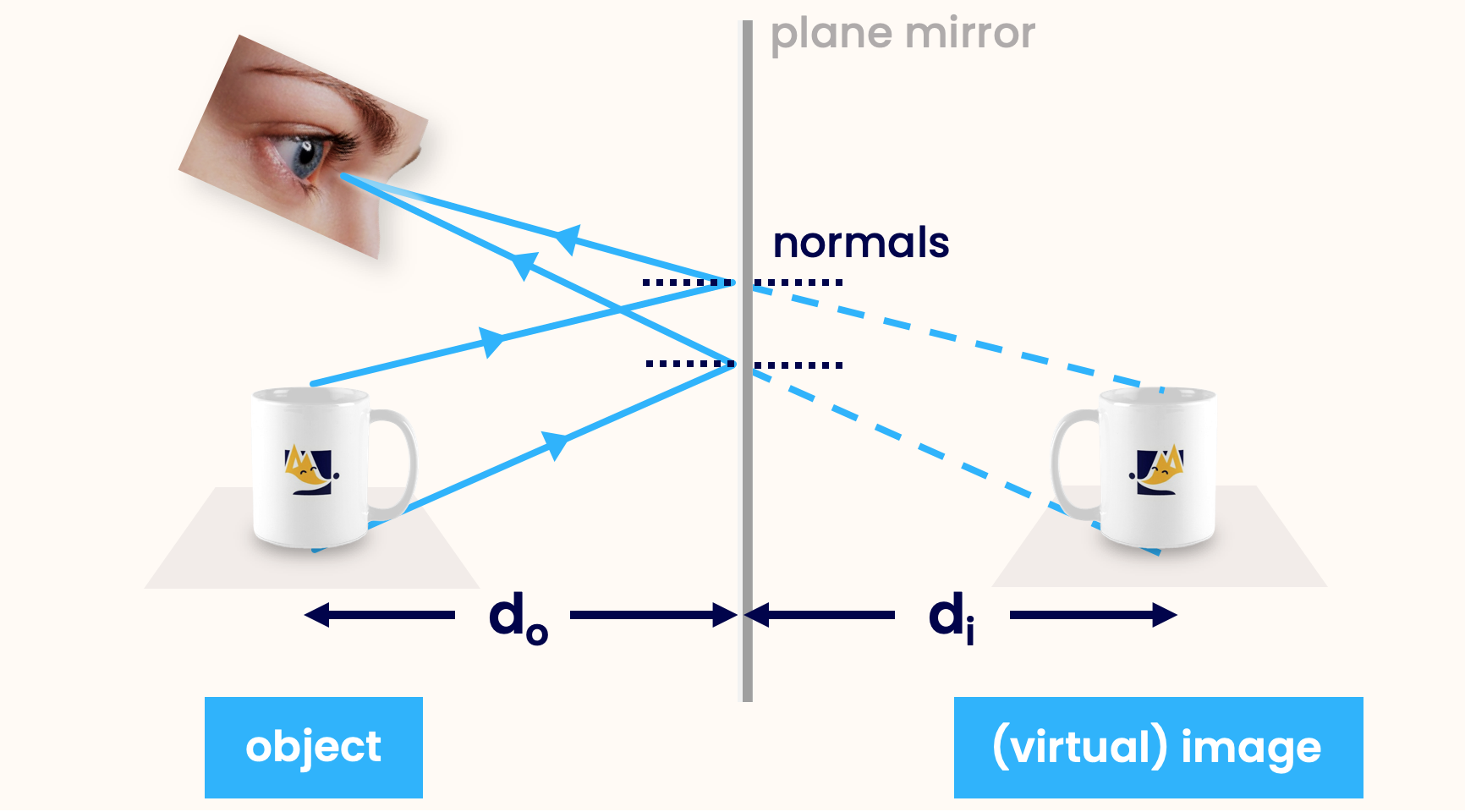• The distance between the object and the mirror ($$d_o$$) is always the same as the distance between the image and the mirror ($$d_i$$): $d_o = d_i$

When I look in the mirror
I don't even recognise myself
Got the heart of a winner
But looking back at me is someone else

Let's do an experiment. I want you to put your hand in front of a mirror and notice two things:

1.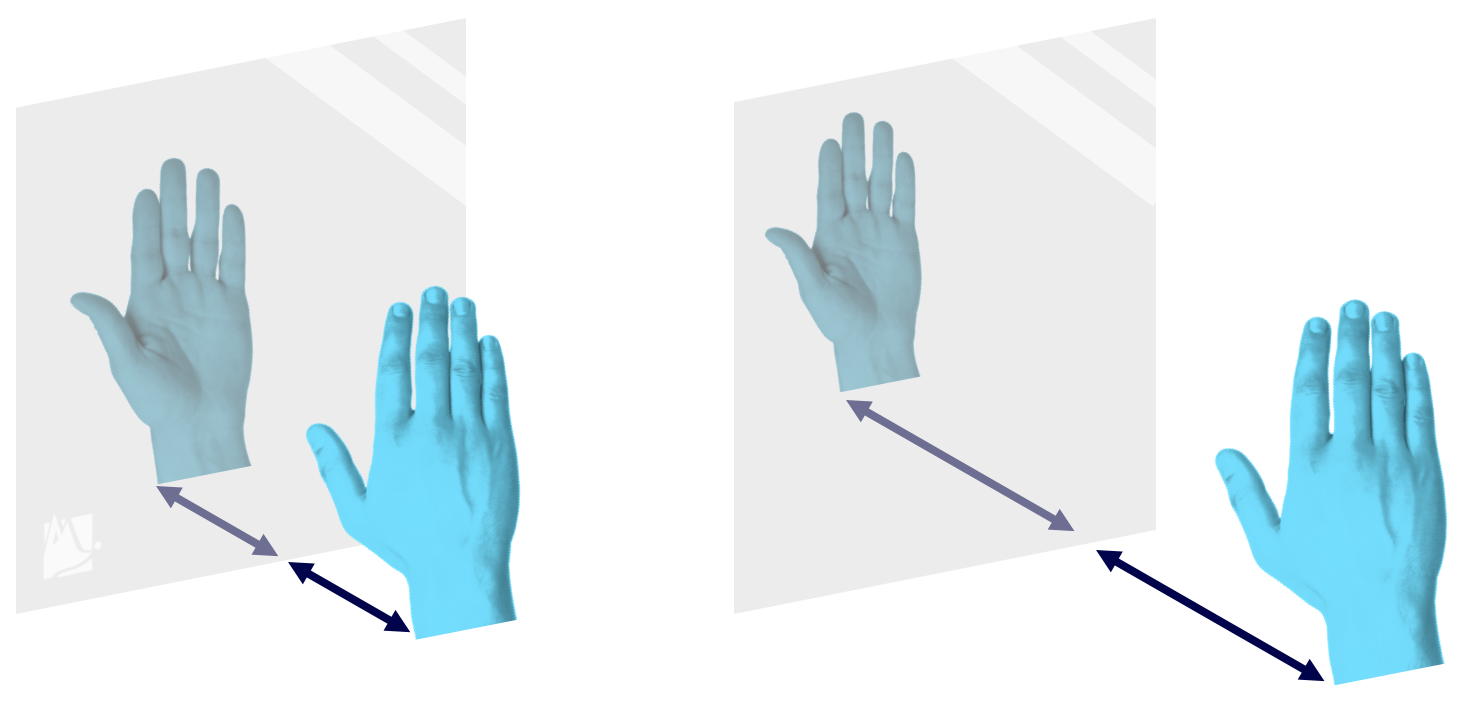The mirror image of your hand is the same distance behind the mirror as your (actual) hand is from the mirror.

(You can test this by moving your hand closer or further away from the mirror. If you hand is 2cm away, the mirror image of you hand will also be 2cm away.)
2.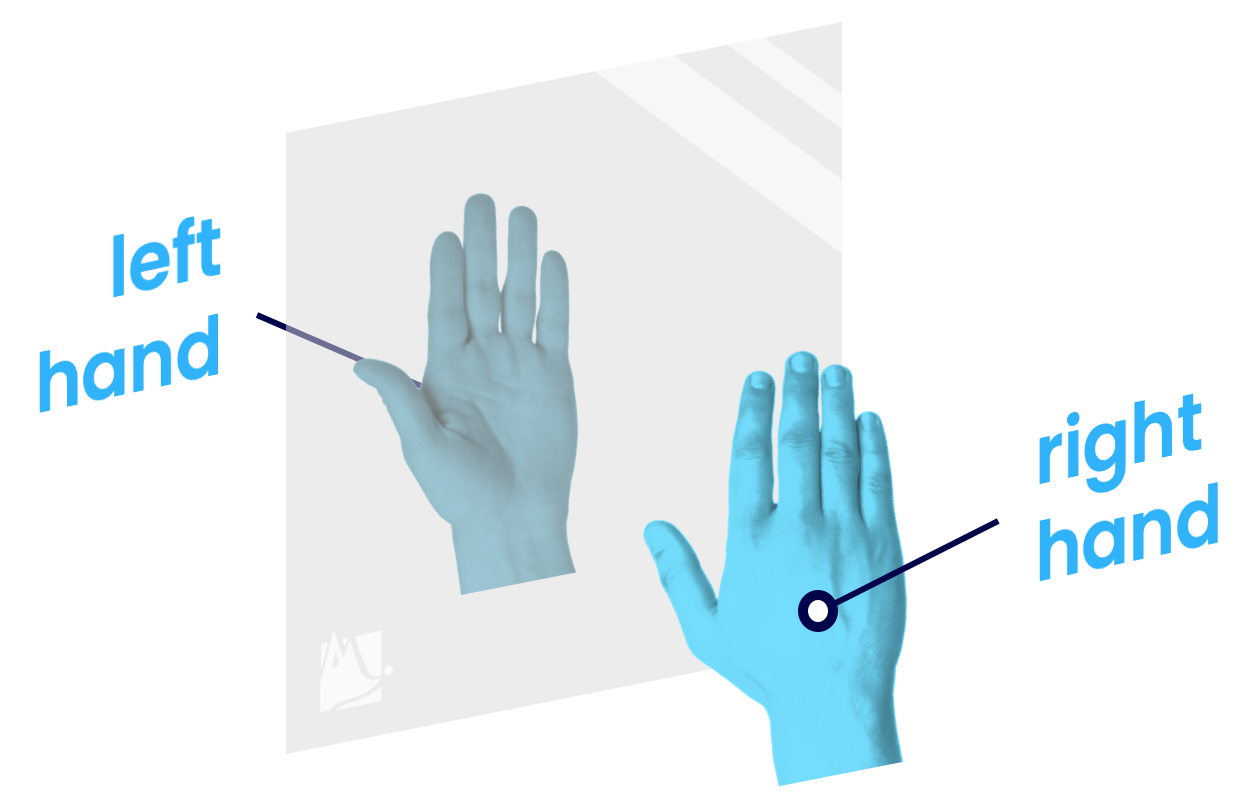Your hand appears flipped left-to-right, but not flipped top-to-bottom. (So if you're holding your right hand up, the mirror image looks like someone else's left hand.) Another way to see this is by holding some text up to a mirror.

If you don't have a mirror near you, try using a black screen: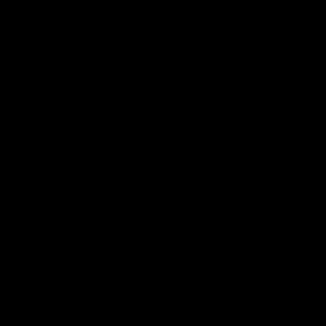It's you!

Let's explain both of these phenomena. We can do this using the Law of Reflection from the last page.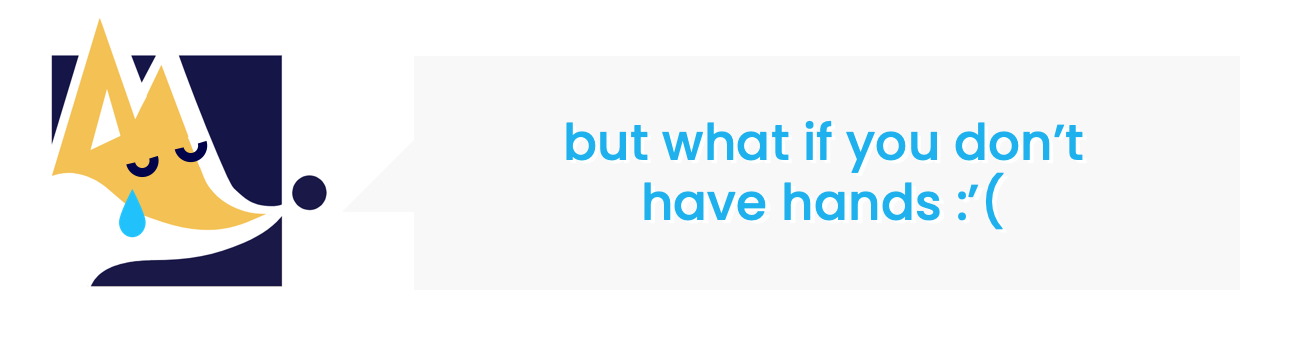Don't worry Philip, it works for paws too!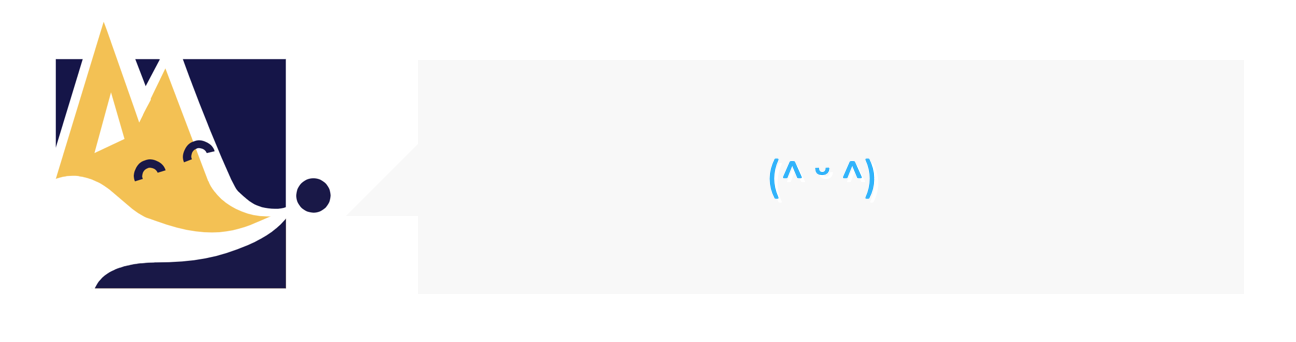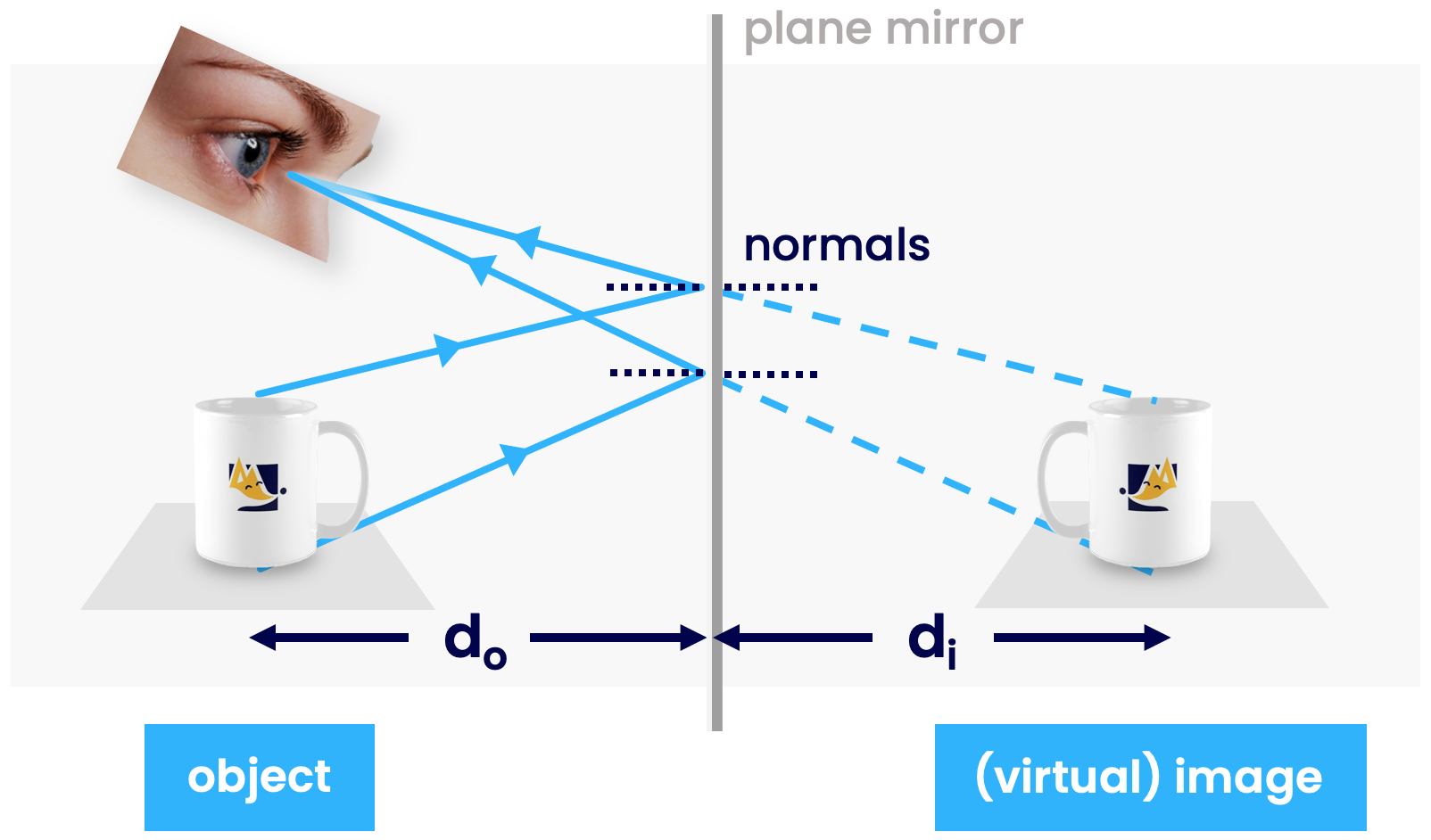This diagram shows light from the top and bottom of an object reflecting off a mirror, and travelling into someone's eye. It is the key to understanding the 'equal distance' phenomenon. It looks a bit complicated, but it'll make sense soon enough! Let's look at it step-by-step:

1. Light reflects off the object (PF mug). It really reflects everywhere off the mug, but we'll just show a couple of rays from the top and bottom to keep it simple.
2. The light rays approach and reflect off the plane mirror. The rays obey the Law of Reflection (they have to, it's a law!)
3. The reflected rays travel into someone's eye.
4. The person sees the object as if it's behind the mirror. This fake object is known as the image.

Why do we see the object as if it's behind the mirror? This is because light travels through space in a straight line — so we have evolved to expect light to always travel this way, even if in reality it has reflected off something in the meantime.

Therefore our brain thinks that the light has travelled along the dotted lines. So objects look like they're behind the mirror! (This is why we "look in the mirror", not "look at the light which the mirror has reflected".)

So why is the image the same distance from the mirror as the (actual) object is? This is because of the law of reflection: i=r. If it instead were i=2r, for example, then the image only be half the distance away (and appear squished).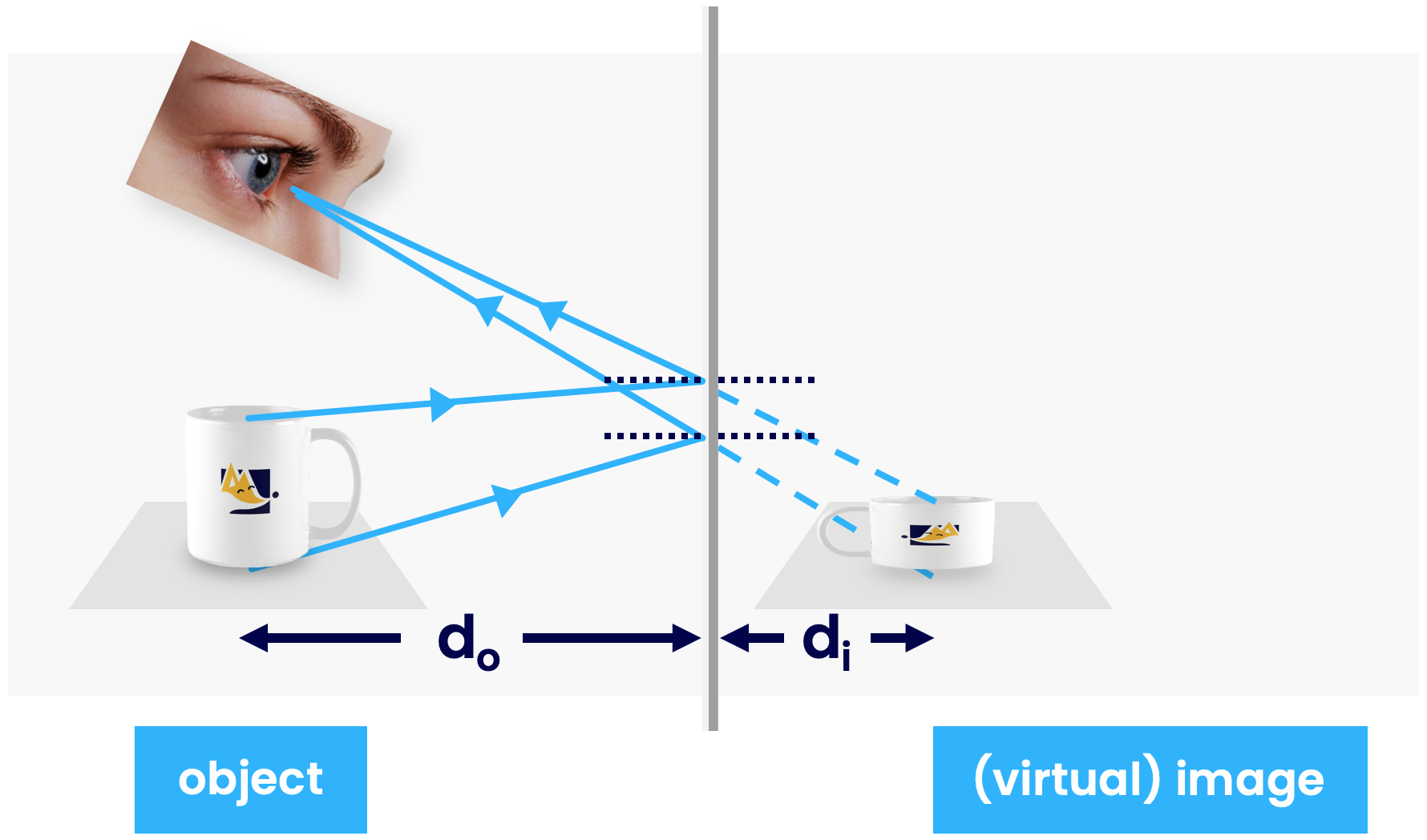This leads us to our second equation: $d_o = d_i$ i.e. distance between object and mirror = distance between image and mirror.

Explaining why objects appear flipped left-to-right is a bit tricky, so don't worry if you don't get it!

To explain this we'll use the fact we've just discovered; $$d_o = d_i$$.$$d_o = d_i$$ isn't just true for an individual object — it is also true for every point on that object. E.g. Your hand obeys $$d_o = d_i$$, but also the tip of each finger obeys $$d_o = d_i$$ too!

Consider the palm of your hand vs the back of your hand. Each of these obeys $$d_o = d_i$$. Since the palm of your hand is closer to the mirror, then $$d_o$$ is smaller for your palm and larger for the back of your hand (say, 3cm vs 4cm). Therefore, $$d_i$$ is also smaller your palm and larger for the back of your hand — the mirror has turned your hand back-to-front!

Of course, it's not just your hand that the mirror has turned back-to-front, it's everything! This is the key. Mirrors don't actually flip things at all — all they do is turn things back-to-front.

Quiz

### Congratulations!

7 of 7 questions completed

+ ⭐️ collected.

• You can change your display name at any time.
• at least 1 letter
• at least 1 number
• at least 8 characters